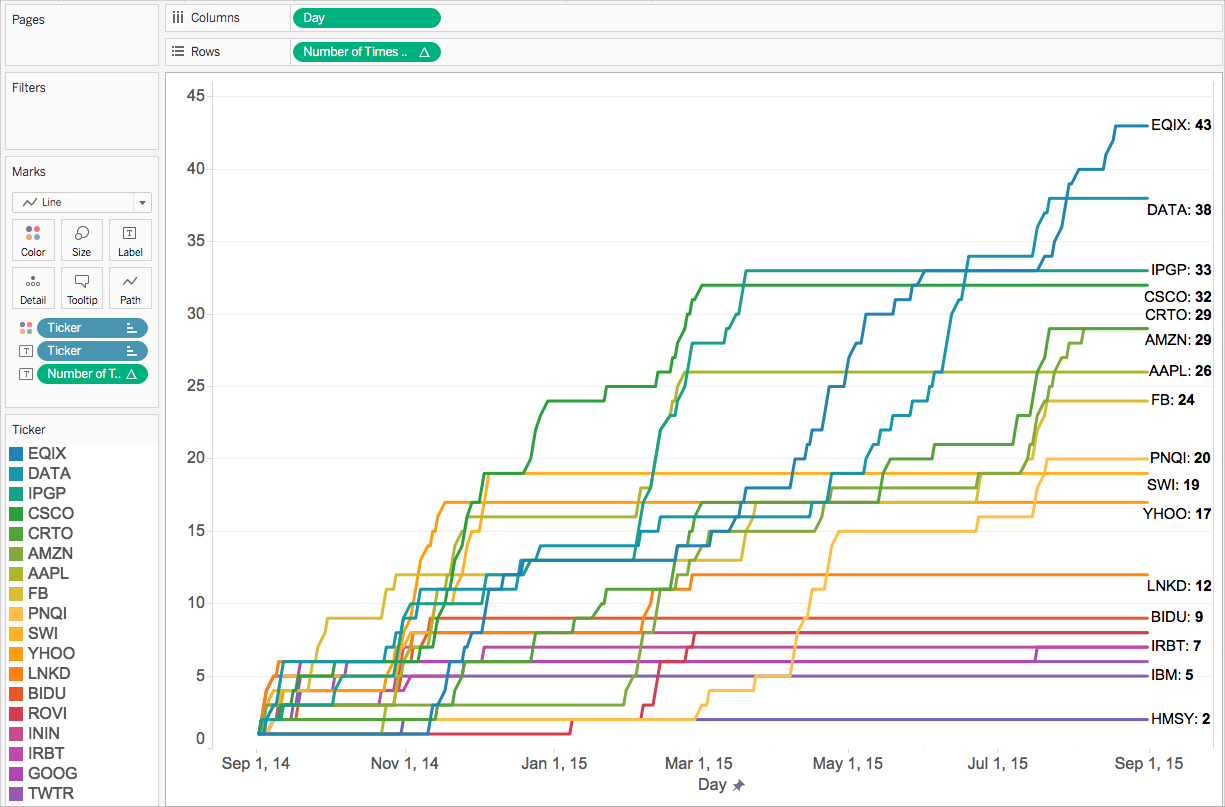The type of calculation you choose depends on the needs of your analysis, the question you want to answer, and the layout of your visualization.

## Which calculation is right for your analysis?

Choosing the type of calculation to use for your analysis is not always easy. When trying to decide, consider the following questions and examples.

Note: This content was originally published on the Tableau Blog. See A Handy Guide to Choosing the Right Calculation for Your Question(Link opens in a new window) to read it.

### Basic expression or table calculation?

##### Question 1: Do you already have all the data values you need on the visualization?

If the answer is yes: You can use a table calculation.

If the answer is no: Use a basic calculation.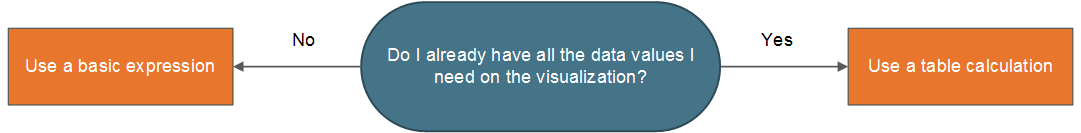##### Example:

Consider the following two visualizations. The visualization on the left is a bar chart that shows the total sales per country/region. The visualization on the right also shows sales per country/region, but sales has been disaggregated.

How could you calculate the 90th percentile of sales for each of these visualizations?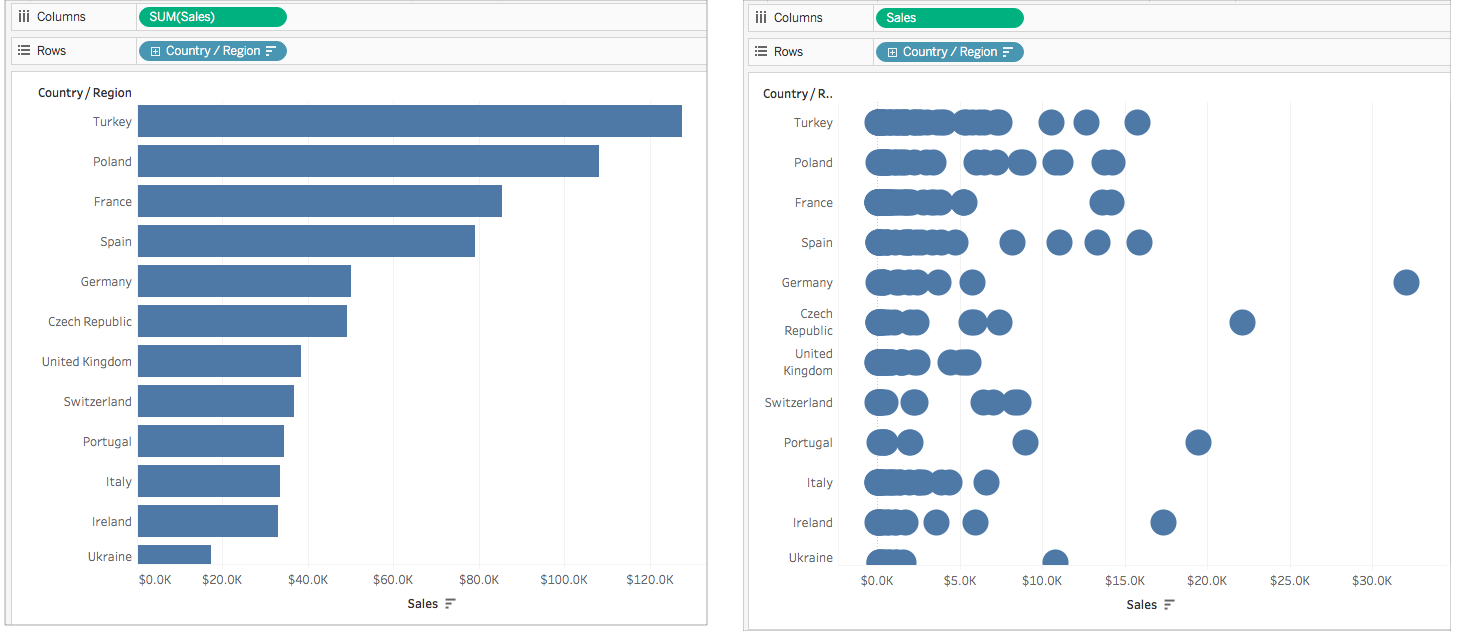The bar chart on the left is aggregated by SUM. Therefore, there is not enough detail in this view to use a table calculation. You can use a basic aggregate expression to calculate the 90th percentile of sales for each country in this example using the following formula:

`PERCENTILE([Sales], .90)`

This results in a value for 90th percentile per country as a label for each bar.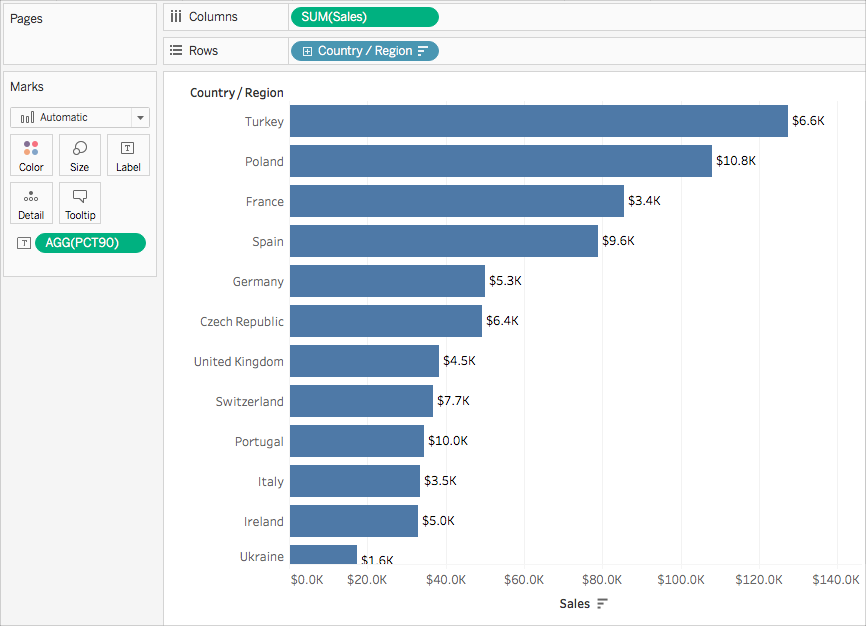However, the chart on the left includes a data value for every sales order. A larger distribution and outliers are shown. There is enough detail in the view to use a table calculation.

You can calculate the 90th percentile of sales for each country by using a distribution band (equivalent to a table calculation). There is more context in this visualization.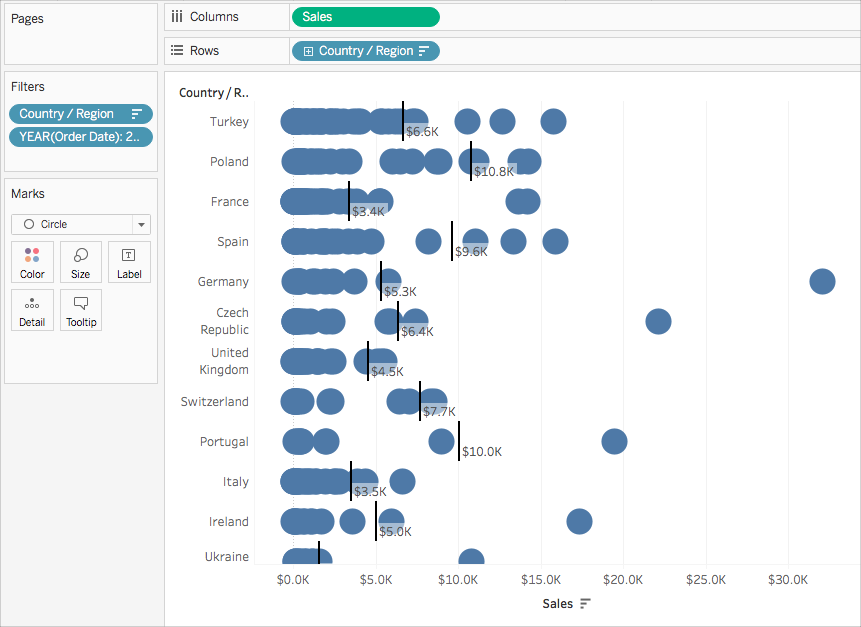Both calculations achieve the same values, but the insights you gather from each differ based on the level of detail (the amount of data) in the visualization.

### Basic expression or Level of Detail (LOD) expression?

If you don't have all the data you need on the visualization, you need your calculation to be passed through to the data source. This means you must use a basic calculation or an LOD Expression.

##### Question 2: Does the granularity of your question match either the granularity of the visualization or the granularity of the data source?

If the answer is yes: Use a basic expression.

If the answer is no: Use a Level of Detail (LOD) expression.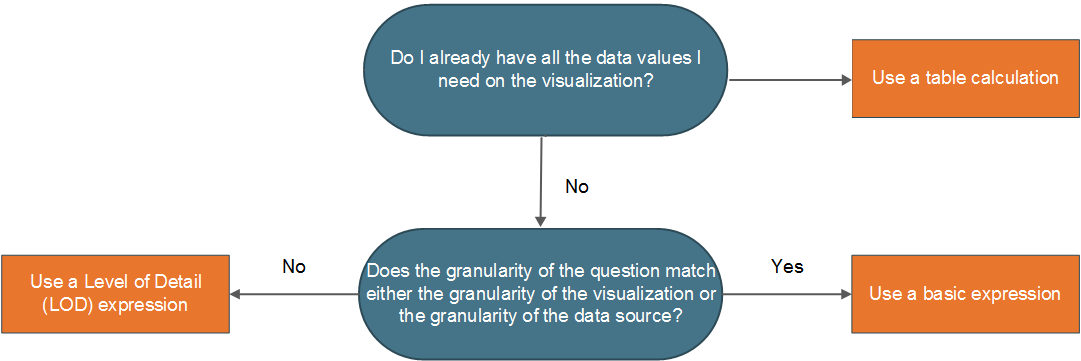##### Example

Consider the following visualization. It shows the 90th percentile of sales for all orders in each country.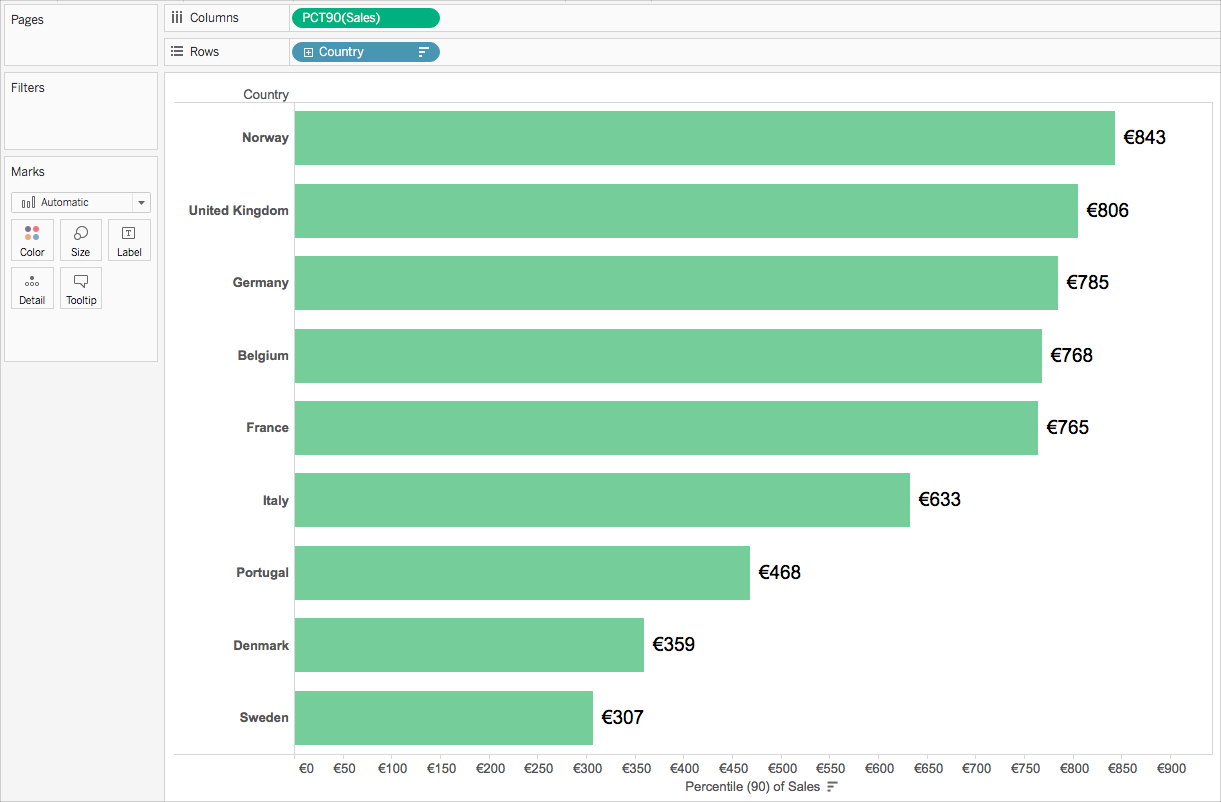This example uses the Sample-Superstore data source that comes with Tableau. If you are familiar with the Sample-Superstore data source, you might know that there is one row of data per Order ID. Therefore, the granularity of the data source is Order ID. The granularity of the visualization, however, is Country.

If you want to know what the 90th percentile value of sales is for orders in each country at the order ID level of granularity, you can use the following LOD expression:

` {INCLUDE [Order ID] : SUM([Sales])}`

You can then change the field to aggregate at the 90th percentile in the view.

To do so, click the field drop-down and select Measure > Percentile > 90.

The following diagram demonstrates how the LOD Expression works in this case: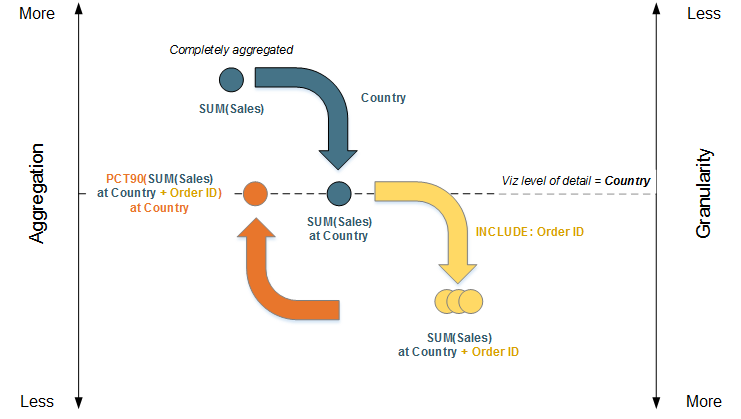1. The data starts completely aggregated at SUM(Sales) and then moves down to the Country level of detail: SUM(Sales) at Country.

2. The LOD calculation is applied and the data gains more granularity: SUM(Sales) at Country + Order ID.

3. The LOD calculation is aggregated to the 90th percentile: PCT90(SUM(Sales) at Country + Order ID)

The result is as follows: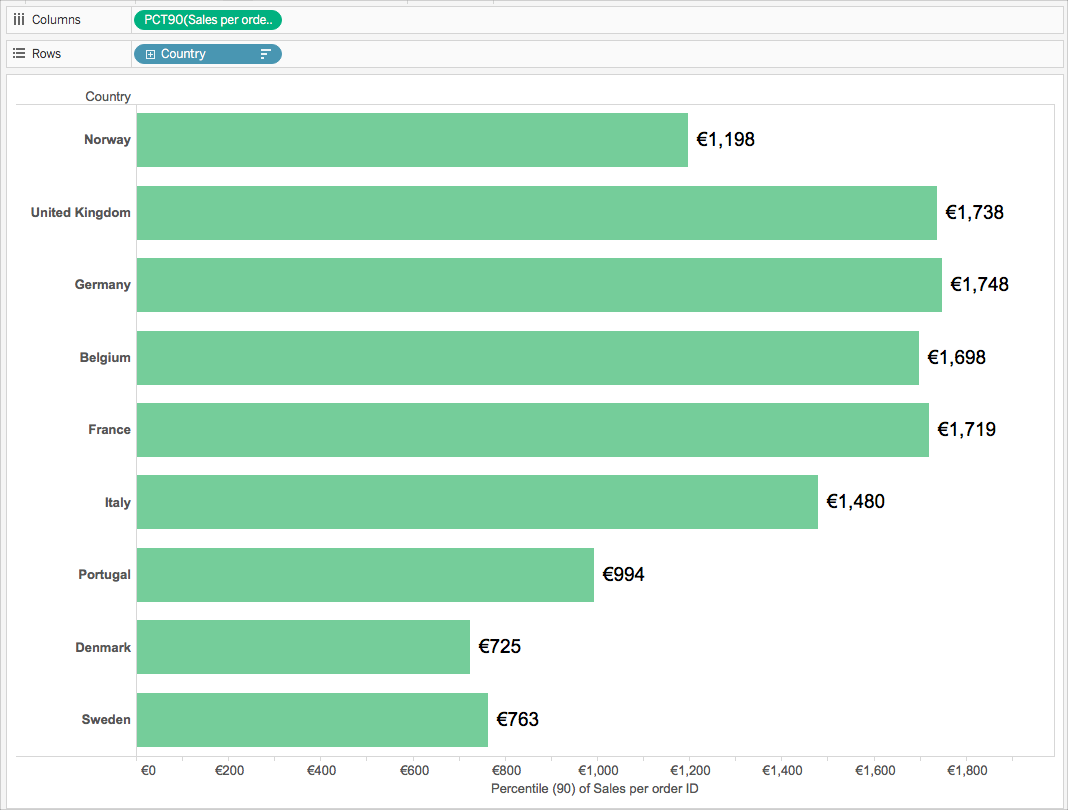### Table calculation or Level of Detail (LOD) expression?

When choosing between a table calculation or an LOD calculation, the process is very similar to choosing between a table calculation and a basic expression. Ask yourself the following questions:

Do you already have all the data values you need on the visualization?

• If the answer is Yes, then use a table calculation.

• If the answer is No, then ask yourself: Does the granularity of the question match either the granularity of the visualization or the granularity of the data source? If the answer is No, then use an LOD calculation.### Table calculations only

There are some scenarios where only a table calculation will do. These include:

• Ranking

• Recursion (e.g. cumulative totals)

• Moving calculations (e.g. rolling averages)

• Inter-row calculations (e.g. period vs. period calculations)

If your analysis requires any of these scenarios, use a table calculation.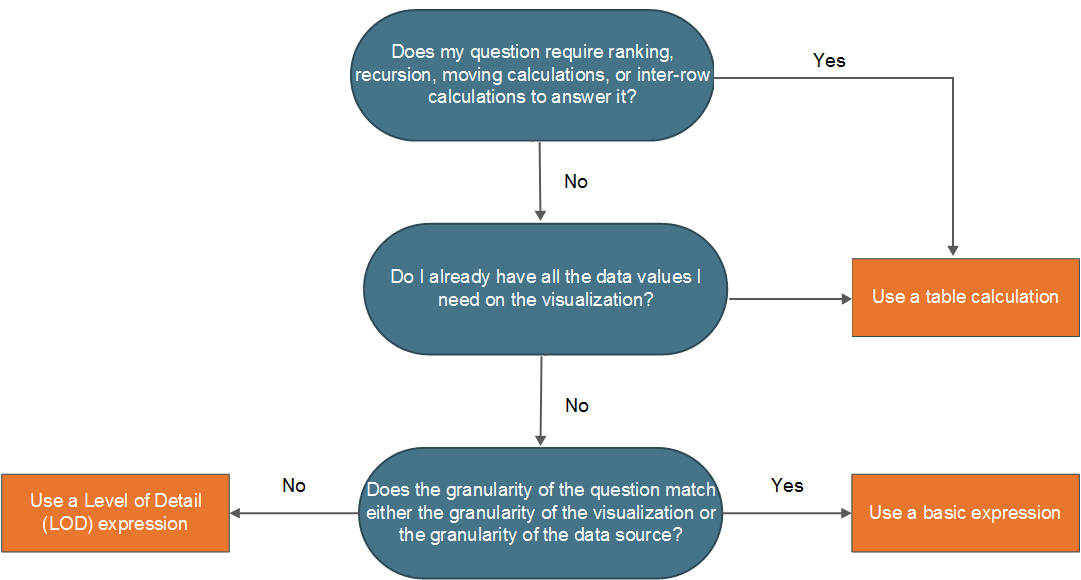##### Example

Consider the following visualization. It shows the average closing price for several stocks between September 2014 and September 2015.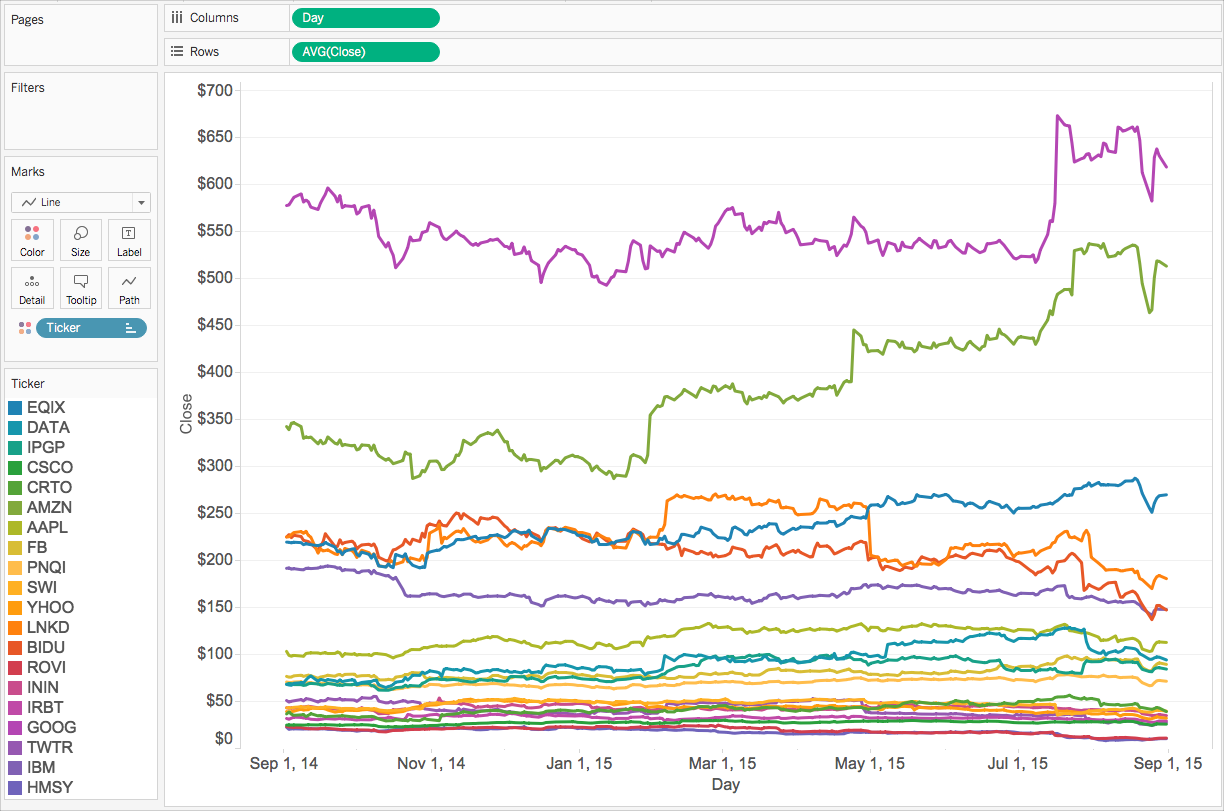If you want to see the number of times the closing price exceeded its record close value to date, you must use a table calculation, specifically a recursive calculation.

Why? Because table calculations can output multiple values for each partition of data (cell, pane, table), while basic and LOD expressions can only output a single value for each partition or grouping of data.

To calculate the number of times the closing price exceeded its record closing price for each stock, there are a few steps you need to take.

1. You need to consider all the previous values before to tell if you have reached a new maximum close value. You can do this with a RUNNING_MAX function. For example, consider the following calculation computed using Day (across the table), titled Record to Date:

`RUNNING_MAX(AVG([Close]))`

2. Next, you can flag the days when the record was broken using the following calculation computed using Day (across the table), titled Count Days Record Broken:

`IF AVG([Close]) = [Record to Date] THEN 1 ELSE 0 END`

3. Finally, you can count these days using the following calculation computed using Day (across the table):

`RUNNING_SUM([Count Days Record Broken])`

When you add the final calculated field to the view in place of Avg(Close), you get something like this: